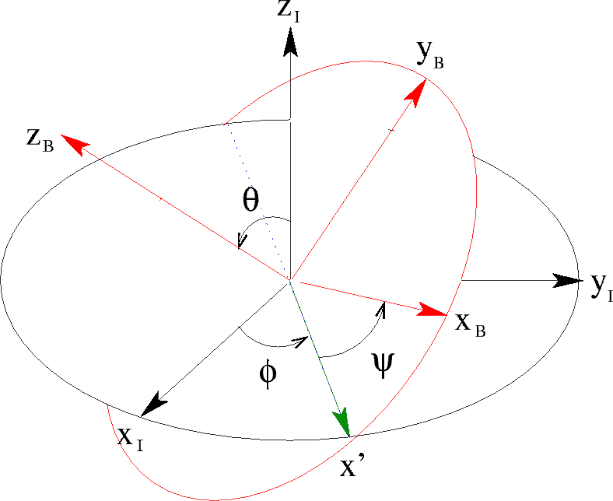# Rotation matrix and rotation of coordinate system

• A
• Kashmir

#### KashmirIf we change the orientation of a coordinate system as shown above, (the standard eluer angles , ##x_1y_1z_1## the initial configuration and ##x_by _b z_b## the final one), then the formula for the coordinates of a vector in the new system is given by

##x'=Ax##
where ##A=\left[\begin{array}{ccc}\cos \psi \cos \phi-\cos \theta \sin \phi \sin \psi & \cos \psi \sin \phi+\cos \theta \cos \phi \sin \psi & \sin \psi \sin \theta \\ -\sin \psi \cos \phi-\cos \theta \sin \phi \cos \psi & -\sin \psi \sin \phi+\cos \theta \cos \phi \cos \psi & \cos \psi \cdot \sin \theta \\ \sin \theta \sin \phi & -\sin \theta \cos \phi & \cos \theta\end{array}\right]##

We observe that the matrix is orthogonal with determinant ##+1## so it's a rotation matrix.
So its effect is to rotate a vector,hence ##x'## will be nothing but ##x## rotated.

So if ##A## represents a clockwise rotation of vectors by ##α## cannot I say that actually the coordinate system has rotated anticlockwise by the same amount ##α##?

•Kashmir
In short, yes. For a bit more details search for the concept of active and passive transformations.
thank you so much :) .